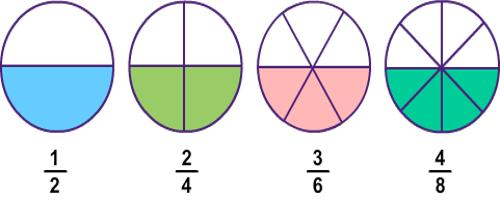Identifying Equivalent Fractions

Equivalent means “equal”. Therefore, equivalent fractions are fractions that are equal in value. Equivalent fractions are fractions that have the same value or represent the same part of an object. If a pie is cut into two pieces, each piece is also one-half of the pie. If a pie is cut into 4 pieces, then two pieces represent the same amount of pie that 1/2 did. We say that 1/2 is equivalent to 2/4.

• You can make equivalent fractions by multiplying or dividing both top and bottom by the same amount.
• You only multiply or divide, never add or subtract, to get an equivalent fraction.
• Only divide when the top and bottom stay as whole numbers.

If the numerator and denominator of a fraction are multiplied (or divided) by the same nonzero number, then the resulting fraction is equivalent to the original fraction.Fractions are determined to be equivalent by multiplying the numerator and denominator of one fraction by the same number. This number should be such that the numerators will be equal after the multiplication. For example if we compare 1/2 and 2/4, we would multiply 1/2 by 2/2 which would result in 2/4 so they are equivalent.

To compare 1/2 and 3/7 we would multiply 1/2 by 3/3 to produce 3/6. Since 3/6 is not the same as 3/7, the fractions are not equivalent.

• Fractions equivalent to 1/2 are 2/4, 3/6, 4/8, 5/10, 6/12 …
• Fractions equivalent to 1/3 are 2/6, 3/9, 4/12, 5/15, …
• Fractions equivalent to 1/4 are 2/8, 3/12, 4/16, 5/20, …
• Fractions equivalent to 1/5 are 2/10, 3/15, 4/20, 5/25, …
• Fractions equivalent to 2/5 are 4/10, 6/15, 8/20, 10/25, …

Multiplying the numerator and the denominator of a fraction by the same nonzero whole number will change that fraction into an equivalent fraction, but it will not change its value. Equivalent fractions may look different, but they have the same value.

Equivalent fractions are different fractions that name the same number. The numerator and the denominator of a fraction must be multiplied by the same nonzero whole number in order to have equivalent fractions.

Information Source: